Select Page

# Answers for MCQ CBSE 12 Science Maths Differential Equations in English

Answers for MCQ CBSE 12 Science Maths Differential Equations in English to enable students to get Answers in a narrative video format for the specific question.

Expert Teacher provides Answers for MCQ CBSE 12 Science Maths Differential Equations through Video Answers in English language. This video solution will be useful for students to understand how to write an answer in exam in order to score more marks. This teacher uses a narrative style for a question from Differential Equations not only to explain the proper method of answering question, but deriving right answer too.

Please find the question below and view the Answer in a narrative video format.

Question:

## Similar Questions from CBSE, 12th Science, Maths, Differential Equations

Question 1 : Write the differential equation formed from the equation y = mx + c, where m and c are arbitrary constants.

Question 2 : If m and n are the order and degree, respectively of the differential equation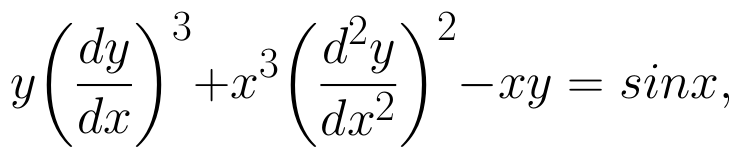then write value of m+n. (View Answer Video)

Question 3 : Find the particular solution of the differential equation :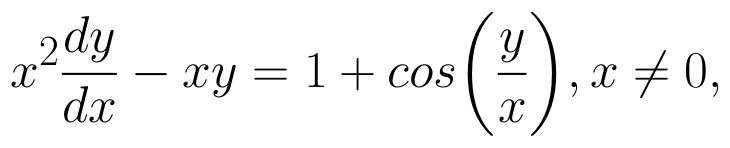when x = 1,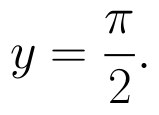(View Answer Video)

Question 4 : Find the general solution of differential equation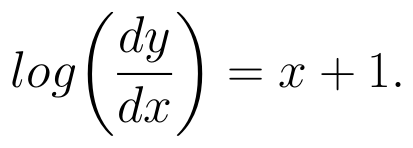(View Answer Video)

Question 5 : Find the particular solution of the differential equation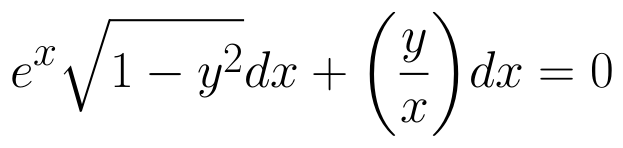given that y = 1 when x = 0. (View Answer Video)

### Inverse Trigonometric Functions

Question 1 : Write the value of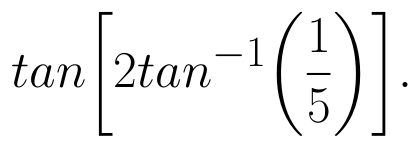(View Answer Video)

Question 2 : Solve for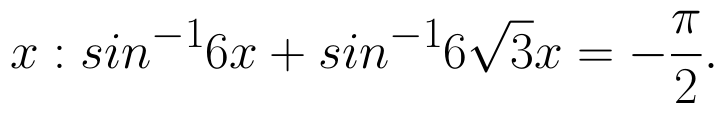(View Answer Video)

Question 3 : What is principal value of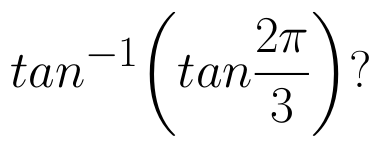(View Answer Video)

Question 4 : Write the principal value of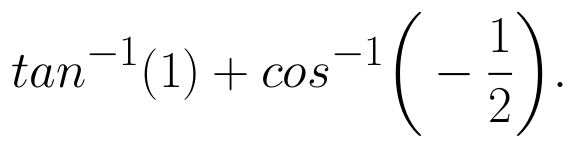(View Answer Video)

Question 5 : Solve for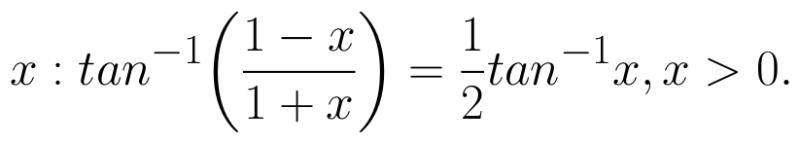(View Answer Video)

### Three Dimensional Geometry

Question 1 : Find the equation of the plane passing through the point (-1, 3, 2) and perpendicular to each of the planes x + 2y + 3z = 5 and 3x + 3y + z = 0. (View Answer Video)

Question 2 : A line passes through the point with position vector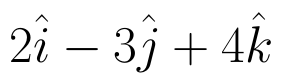and makes angles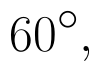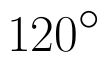and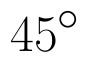with x, y and z-axis respectively. Find the equation of the line in the Cartesian form. (View Answer Video)

Question 3 : Find the intercepts cut off by the plane 2x + y - z = 5. (View Answer Video)

Question 4 : If lines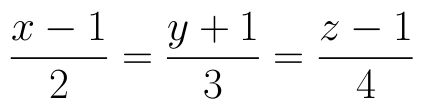and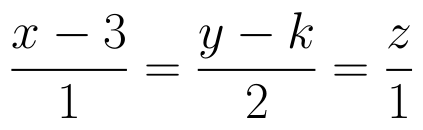intersect, then find the value of k and hence find the equation of plane containing these lines. (View Answer Video)

Question 5 : Find the distance between the point (-1, -5, -10) and the point of intersection of line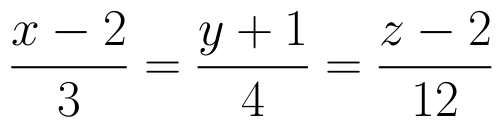and plane

### Vector Algebra

Question 1 : Find the angle between two vectors a and b with magnitudes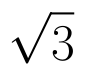and 2 respectively, having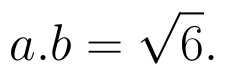(View Answer Video)

Question 2 : Find the vector quantities from the following:
(i) 10 kg          (ii) 2 m north-west          (iii)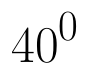(iv) 40 W         (v)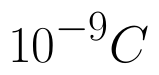(vi)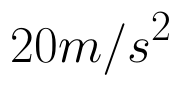(View Answer Video)

Question 3 : Find |a| and |b|, if (a + b).(a - b) = 8 and |a| = 8|b|. (View Answer Video)

Question 4 : Find a unit vector parallel to the sum of vectors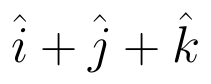and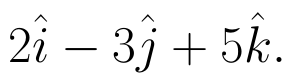(View Answer Video)

Question 5 : Compute the magnitude of the following vectors: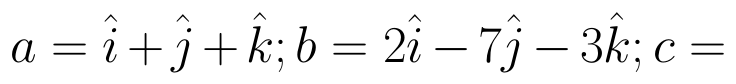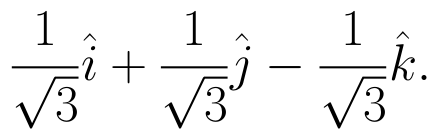(View Answer Video)